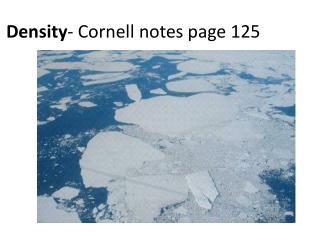# Density - Cornell notes page 125 - PowerPoint PPT PresentationDownload PresentationDensity - Cornell notes page 125

Density - Cornell notes page 125Download Presentation## Density - Cornell notes page 125

- - - - - - - - - - - - - - - - - - - - - - - - - - - E N D - - - - - - - - - - - - - - - - - - - - - - - - - - -
##### Presentation Transcript

1. Density- Cornell notes page 125

2. Density • Physical property of matter • amount of matter in a given amount of space. • (amount of matter is called “mass,” and amount of space an object takes up is called “volume”) • Density= Mass/Volume • http://www.youtube.com/watch?v=FSupQZQqaPk

3. Density • How do we find mass? • How do we find volume?

4. Density • How do we find mass? • triple beam balance, scale • How do we find volume? • multiply length, width, and height • use displacement method • 1 mL= 1 cm3 (centimeter cubed/cubic centimeter= cc)

5. Density • Water has a given density of 1g/mL • Things that are less dense will float on water. • Things that are more dense will sink.

6. Back to the Egg… 1. Did the egg change? 2. In Cup #1 is the egg more dense or less dense than the liquid? 3. In Cup #2 is the egg more dense or less dense than the liquid? 4. Is the liquid in Cup #1 more dense or less dense than Cup #2?

7. Density Practice Copy and complete on page 125 in science notebook

8. Density Practice Use the data below to calculate the density of each unknown substance. Then use the density chart to determine the identify of each substance. Mass Volume Density Substance(g) (cm3) (g/cm3) • 171 15 ______ _______ • 108 40 ______ _______ • 475 250 ______ _______ • 680 1000 ______ _______

9. Density Practice Use the data below to calculate the density of each unknown substance. Then use the density chart to determine the identify of each substance. Mass Volume Density Substance(g) (cm3) (g/cm3) • 171 15 11.4g/cm3Lead • 108 40 2.7g/cm3Aluminum • 475 250 1.9g/cm3Bone • 680 1000 0.68g/cm3Gasoline